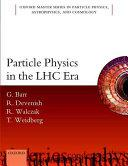# Particle Physics In The Lhc Era

Free shipping (Guaranteed delivery 3-5 business days for In-Stock products)

## ISBN details

• ISBN 10: 0198748566
• ISBN 13: 9780198748564

## Overview

This text gives an introduction to particle physics at a level accessible to advanced undergraduate students. It is based on lectures given to 4th year physics students over a number of years, and reflects the feedback from the students. The aim is to explain the theoretical and experimental basis of the Standard Model (SM) of Particle Physics with the simplest mathematical treatment possible. All the experimental discoveries that led to the understanding of the SM relied on particle detectors and most of them required advanced particle accelerators. A unique feature of this book is that it gives a serious introduction to the fundamental accelerator and detector physics, which is currently only available in advanced graduate textbooks. The mathematical tools that are required such as group theory are covered in one chapter. A modern treatment of the Dirac equation is given in which the free particle Dirac equation is seen as being equivalent to the Lorentz transformation. The idea of generating the SM interactions from fundamental gauge symmetries is explained. The core of the book covers the SM. The tools developed are used to explain its theoretical basis and a clear discussion is given of the critical experimental evidence which underpins it. A thorough account is given of quark flavour and neutrino oscillations based on published experimental results, including some from running experiments. A simple introduction to the Higgs sector of the SM is given. This explains the key idea of how spontaneous symmetry breaking can generate particle masses without violating the underlying gauge symmetry. A key feature of this book is that it gives an accessible explanation of the discovery of the Higgs boson, including the advanced statistical techniques required. The final chapter gives an introduction to LHC physics beyond the standard model and the techniques used in searches for new physics. There is an outline of the shortcomings of the SM and a discussion of possible solutions and future experiments to resolve these outstanding questions.

## Other Details

• Publisher: Oxford University Press,
• Language: Eng
• Edition: 1
• Date Published: 2016
• Authors: Barr, G. (giles), Devenish, Robin, Walczak, R. (roman), Weidberg, T. (tony)